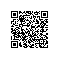# oracle 10g rac客户端配置

# tnsnames.ora Network Configuration File: C:\oracle\product\10.2.0\client_1\NETWORK\ADMIN\tnsnames.ora
# Generated by Oracle configuration tools.

RACWEB =
(DESCRIPTION =
(ADDRESS = (PROTOCOL = TCP)(HOST = 192.168.1.154)(PORT = 1521))
(ADDRESS = (PROTOCOL = TCP)(HOST = 192.168.1.155)(PORT = 1521))
(CONNECT_DATA =
(SERVER = DEDICATED)
(SERVICE_NAME = racweb.lilianwang.com)
)
)

RACWEB2 =
(DESCRIPTION =
(ADDRESS = (PROTOCOL = TCP)(HOST = 192.168.1.155)(PORT = 1521))
(CONNECT_DATA =
(SERVER = DEDICATED)
(SERVICE_NAME = racweb.lilianwang.com)
(INSTANCE_NAME = racweb2)
)
)

RACWEB1 =
(DESCRIPTION =
(ADDRESS = (PROTOCOL = TCP)(HOST = 192.168.1.154)(PORT = 1521))
(CONNECT_DATA =
(SERVER = DEDICATED)
(SERVICE_NAME = racweb.lilianwang.com)
(INSTANCE_NAME = racweb1)
)
)

LISTENERS_RACWEB =
(ADDRESS = (PROTOCOL = TCP)(HOST = 192.168.1.154)(PORT = 1521))
(ADDRESS = (PROTOCOL = TCP)(HOST = 192.168.1.155)(PORT = 1521))
)

RACWEBDB =
(DESCRIPTION =
(ADDRESS = (PROTOCOL = TCP)(HOST = 192.168.1.154)(PORT = 1521))
(ADDRESS = (PROTOCOL = TCP)(HOST = 192.168.1.155)(PORT = 1521))
(CONNECT_DATA =
(SERVER = DEDICATED)
(SERVICE_NAME = racwebdb.lilianwang.com)
(FAILOVER_MODE =
(TYPE = SELECT)
(METHOD = BASIC)
(RETRIES = 180)
(DELAY = 5)
)
)
)

RACDB1 =
(DESCRIPTION =
(ADDRESS = (PROTOCOL = TCP)(HOST = 192.168.1.245)(PORT = 1521))
(CONNECT_DATA =
(SERVER = DEDICATED)
(SERVICE_NAME = racdb)
(INSTANCE_NAME = racdb1)
)
)

LISTENERS_RACDBIDC =
(ADDRESS = (PROTOCOL = TCP)(HOST = 192.168.1.115)(PORT = 1521))
(ADDRESS = (PROTOCOL = TCP)(HOST = 192.168.1.116)(PORT = 1521))
)

SNMP_SD =
(DESCRIPTION =
(ADDRESS = (PROTOCOL = TCP)(HOST = 192.168.0.63)(PORT = 1521))
)
(CONNECT_DATA =
(SERVICE_NAME = snmp)
)
)

RACDB =
(DESCRIPTION =
(ADDRESS = (PROTOCOL = TCP)(HOST = 192.168.1.245)(PORT = 1521))
(ADDRESS = (PROTOCOL = TCP)(HOST = 192.168.1.246)(PORT = 1521))
(CONNECT_DATA =
(SERVER = DEDICATED)
(SERVICE_NAME = racdb)
)
)

RACDBIDC2_61.50.143.110 =
(DESCRIPTION =
(ADDRESS = (PROTOCOL = TCP)(HOST = 61.50.143.110)(PORT = 61521))
(CONNECT_DATA =
(SERVER = DEDICATED)
(SERVICE_NAME = racdbidc)
(INSTANCE_NAME = racdbidc2)
)
)

RAC136WAN =
(DESCRIPTION =
(ADDRESS = (PROTOCOL = TCP)(HOST = 61.50.143.110)(PORT = 1521))
)
(CONNECT_DATA =
(SID = racdb2)
(SERVER = DEDICATED)
)
)

GEGW_SD =
(DESCRIPTION =
(ADDRESS = (PROTOCOL = TCP)(HOST = 192.168.0.13)(PORT = 1521))
)
(CONNECT_DATA =
(SERVICE_NAME = gegw)
)
)

GEGWBAK =
(DESCRIPTION =
(ADDRESS = (PROTOCOL = TCP)(HOST = 192.168.13.42)(PORT = 1521))
)
(CONNECT_DATA =
(SERVICE_NAME = gegwbak)
)
)

RACDBIDC =
(DESCRIPTION =
(ADDRESS = (PROTOCOL = TCP)(HOST = 192.168.1.115)(PORT = 1521))
(ADDRESS = (PROTOCOL = TCP)(HOST = 192.168.1.116)(PORT = 1521))
(CONNECT_DATA =
(SERVER = DEDICATED)
(SERVICE_NAME = racdbidc)
(FAILOVER_MODE =
(TYPE = SELECT)
(METHOD = BASIC)
(RETRIES = 180)
(DELAY = 5)
)
)
)

LISTENERS_RACDB =
(ADDRESS = (PROTOCOL = TCP)(HOST = 192.168.1.245)(PORT = 1521))
(ADDRESS = (PROTOCOL = TCP)(HOST = 192.168.1.246)(PORT = 1521))
)

GEGW =
(DESCRIPTION =
(ADDRESS = (PROTOCOL = TCP)(HOST = 192.168.0.13)(PORT = 1521))
)
(CONNECT_DATA =
(SERVICE_NAME = gegw)
)
)

SNMP =
(DESCRIPTION =
(ADDRESS = (PROTOCOL = TCP)(HOST = 192.168.0.63)(PORT = 1521))
)
(CONNECT_DATA =
(SERVICE_NAME = snmp)
)
)

RACWEB1_123.124.18.198 =
(DESCRIPTION =
(ADDRESS = (PROTOCOL = TCP)(HOST = 123.124.18.198)(PORT = 51521))
)
(CONNECT_DATA =
(SID = racweb1)
(SERVER = DEDICATED)
)
)

RACDBIDC1_61.50.143.110 =
(DESCRIPTION =
(ADDRESS = (PROTOCOL = TCP)(HOST = 61.50.143.110)(PORT = 51521))
(CONNECT_DATA =
(SERVER = DEDICATED)
(SERVICE_NAME = racdbidc)
(INSTANCE_NAME = racdbidc1)
)
)

MIG =
(DESCRIPTION =
(ADDRESS = (PROTOCOL = TCP)(HOST = 192.168.0.15)(PORT = 1521))
)
(CONNECT_DATA =
(SERVICE_NAME = mig)
)
)

AM012 =
(DESCRIPTION =
(ADDRESS = (PROTOCOL = TCP)(HOST = 220.194.52.85)(PORT = 1522))
)
(CONNECT_DATA =
(SERVICE_NAME = am012)
)
)

AM011 =
(DESCRIPTION =
(ADDRESS = (PROTOCOL = TCP)(HOST = 220.194.52.85)(PORT = 1521))
)
(CONNECT_DATA =
(SERVICE_NAME = am011)
)
)

GEGW002 =
(DESCRIPTION =
(ADDRESS = (PROTOCOL = TCP)(HOST = 192.168.13.11)(PORT = 1521))
)
(CONNECT_DATA =
(SERVICE_NAME = gegw002)
)
)

RACDBIDC2 =
(DESCRIPTION =
(ADDRESS = (PROTOCOL = TCP)(HOST = 192.168.1.116)(PORT = 1521))
(CONNECT_DATA =
(SERVER = DEDICATED)
(SERVICE_NAME = racdbidc)
(INSTANCE_NAME = racdbidc2)
)
)

RACDBIDC1 =
(DESCRIPTION =
(ADDRESS = (PROTOCOL = TCP)(HOST = 192.168.1.115)(PORT = 1521))
(CONNECT_DATA =
(SERVER = DEDICATED)
(SERVICE_NAME = racdbidc)
(INSTANCE_NAME = racdbidc1)
)
)

LLPWDB_192.168.1.240 =
(DESCRIPTION =
(ADDRESS = (PROTOCOL = TCP)(HOST = 192.168.1.240)(PORT = 1521))
)
(CONNECT_DATA =
(SID = llpwdb)
(SERVER = DEDICATED)
)
)

EXTPROC_CONNECTION_DATA =
(DESCRIPTION =
(ADDRESS = (PROTOCOL = IPC)(KEY = EXTPROC0))
)
(CONNECT_DATA =
(SID = PLSExtProc)
(PRESENTATION = RO)
)
)

RACDB2 =
(DESCRIPTION =
(ADDRESS = (PROTOCOL = TCP)(HOST = 192.168.1.246)(PORT = 1521))
(CONNECT_DATA =
(SERVER = DEDICATED)
(SERVICE_NAME = racdb)
(INSTANCE_NAME = racdb2)
)
)使用钉钉扫一扫加入圈子
+ 订阅